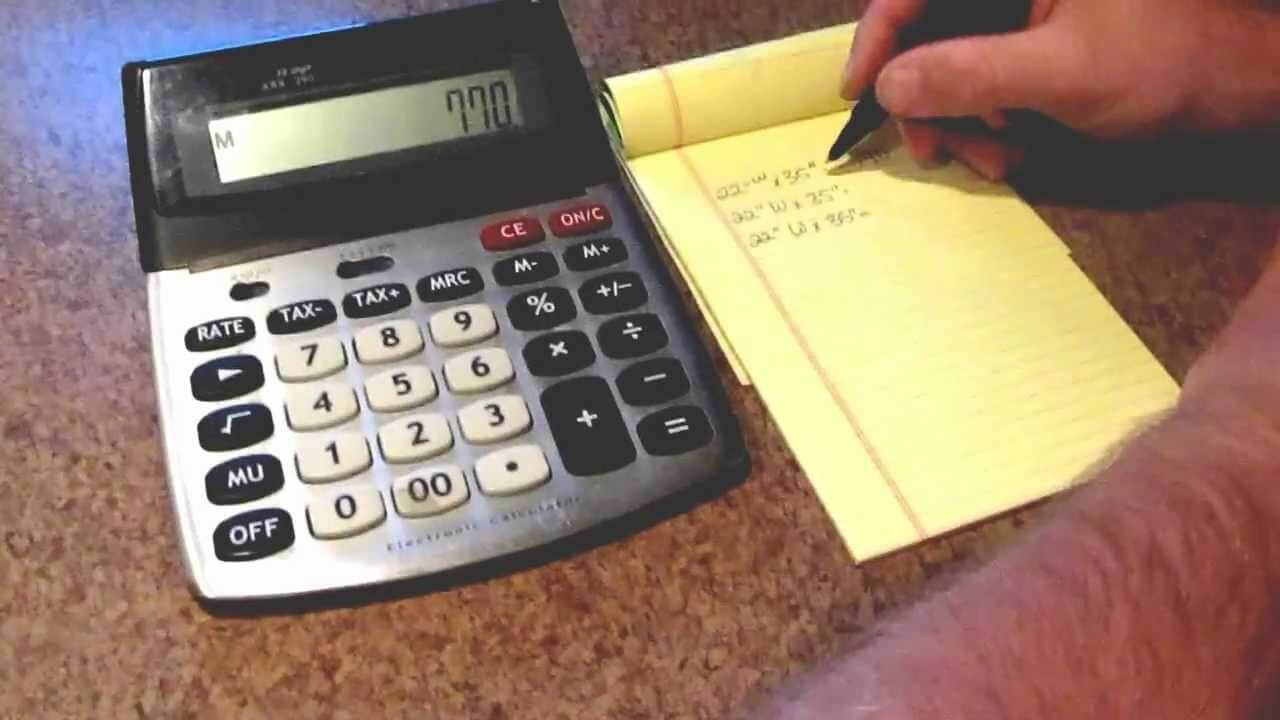Whenever you will read about the properties, you will come across the term square footage as it is an imperial unit that indicates the living area space. It is widely being used in numerous countries like the US, Pakistan, Canada, Bangladesh, Ghana, Myanmar, Singapore, and many others. Thus, it is a well-known term in the real estate industry as people measure the dimensions and indicate the result by using the term square foot. It is widely referred to by its abbreviation which can be either sqft or sq ft.

There are tons of other units of areas to measure space but this one is widely preferred. The reason that it has the term foot or feet in the name is that it is equivalent to the area of a square with sides of a single foot. This is one of the reasons it is used to measure everyday objects and properties making it a vital solution to indicate the overall size of tiny and gigantic objects.

So, whether you are shopping for a carpet or all set to renovate your house, it will come in handy to measure the exact size through this renowned unit. Not to forget to make use of the square feet calculator which will help you to measure anything with utmost convenience. It will also come in handy while you sell, buy, or rent a house as the price is evaluated by the total square foot of the property.

There are some countries that prefer to measure in square meters and square inches. That makes it important to learn the formula of conversion as you will come across a point where it will be useful.

When it is about converting square meters into square feet, then you have to remember that 1 sq. m equals to 10.764 sq ft. Next to that, you have to simply multiply the total number of sq. m by 10.764 and you will get the correct answer which will be the sq ft of the area you’re measuring. Additionally, you can leverage the square feet calculator and do the entire calculation in just a few clicks.

The formula of converting sq into sq ft is effortless as well. A single square foot is equal to 144 square inches. So, the formula to convert will be as follow:

Square feet: square inches / 144

Although the calculation is quite easy but using the square feet calculator can make the process completely hectic-free as all you need is to enter the number of sq in that you want to convert and it will show you the precise answer in no time.

These calculators can be easily accessed by searching for the term in the search engine and you will find the best ones that can help you to measure the square footage of any shape like concrete, gravel, tile, trapezoid, circumference, or anything.

Along with square feet calculator, one should also be familiar with the method of manual calculation so that you can find the exact answer on the go. Once you get to know about the procedure, you will realize that it’s just a piece of cake.

The first thing you will need is a measuring tape or a laser tape. Now that you have got the basic accessory, you can start by measuring the length and width of a chosen space. Make sure that the measured figure is correct otherwise, you will end up with an incorrect measurement.

Once you have got the length and width of a space, you have to multiply both and the answer that you will get is the square footage of the chosen area. But if it’s about different shapes, then the same formula won’t be applied and that’s what actually makes it difficult to measure square feet of a constructed property.

It can be tricky for some to make use of square feet calculator and measure the precise area land of space but there’s an option to hire an appraiser. The reason to hire a professional is that they know about the rules and regulations that are set by the estate. There are some spaces like the basement and garages that are not included in the total square footage of the property and not to forget the difficult formulas for different shapes. A trained person can do this task in just a few hours by making use of online calculators. So, if you are facing difficulty, then you will have to spend a bit of your money, and you are good to go.

These days, it has become essential to have sound knowledge about square feet as the majority of countries prefer the imperial system over the metric system. So, if you have knowledge about converting sq inches and sq meters into sq ft, then it will come in handy anytime. Further, square feet calculator can assist you in measurement as well.